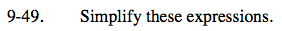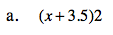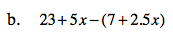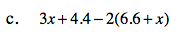Home > CC2MN > Chapter cc29 > Lesson cc29.1.3 > Problem9-49

9-49.
1. Simplify these expressions. Homework Help ✎

1. (x + 3.5)2

2. 23 + 5x − (7 + 2.5x)

3. 3x + 4.4 − 2(6.6 + x)2x + 7Distribute the subtraction sign to the terms inside the parentheses, and then combine like terms. See part (c) for a worked example.3x + 4.4 − 2(6.6 + x)

Remember to distribute the negative inside the parentheses.

3x + 4.4 − 13.2 − 2x

Combine like terms by adding or subtracting the constants.

x − 8.8